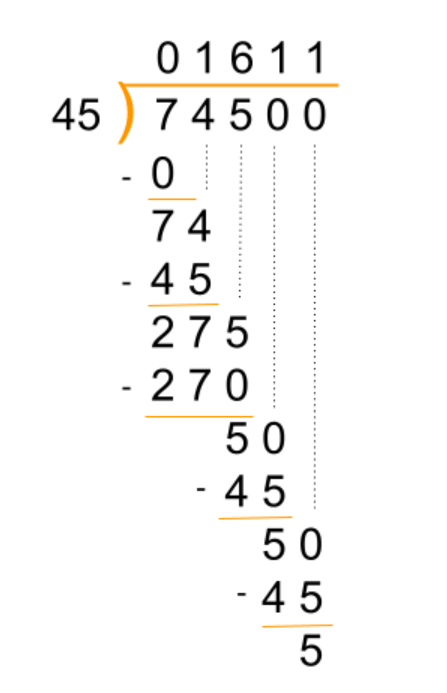# Long Division Calculator

Choose the method and enter the divisor and the dividend in the long division calculator. Hit “Calculate”.## Long Division Calculator

This online long division calculator is used to perform the divisions using remainder and decimal methods with steps.

You can choose the decimal place in this tool. It is also called the remainder and quotient calculator.

## What is the long division?

Long division means applying the division operation on a rather large divisor and dividend. It has the same process as simple division. The division symbol is a little different.

The calculator is used to perform long divisions because they are time-taking and have rather more possibility of error.

## How to perform long divisions?

### Example:

Find the quotient up to 2 decimal places for 725 / 45.

Solution:

1.Put the values in the long division symbol: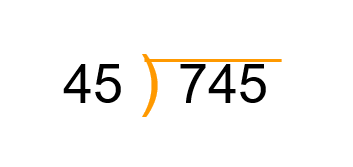2.Put 0 in the quotient value to bring another value down. We do this to make the value dividable (ten-value).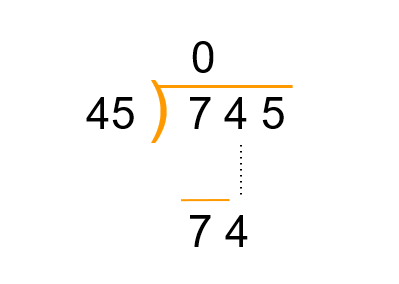3.Put 1 in the quotient to have 45 below 74 as it is the closest.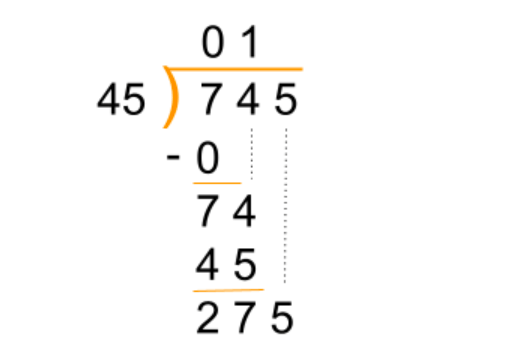4.Put 6 in the quotient and 270 below 275. After subtracting, add and carry down a zero to make it a ten-value.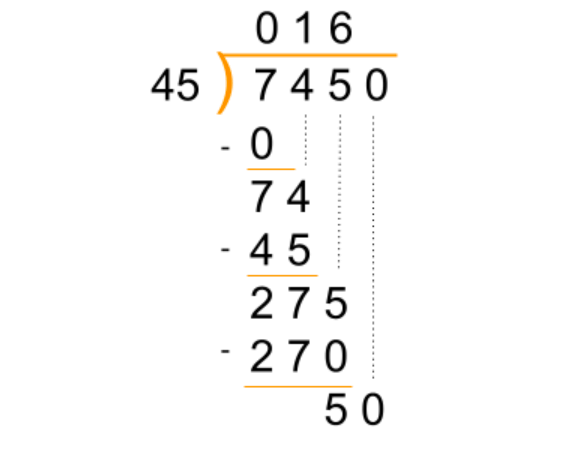5.Solve until two decimal places.